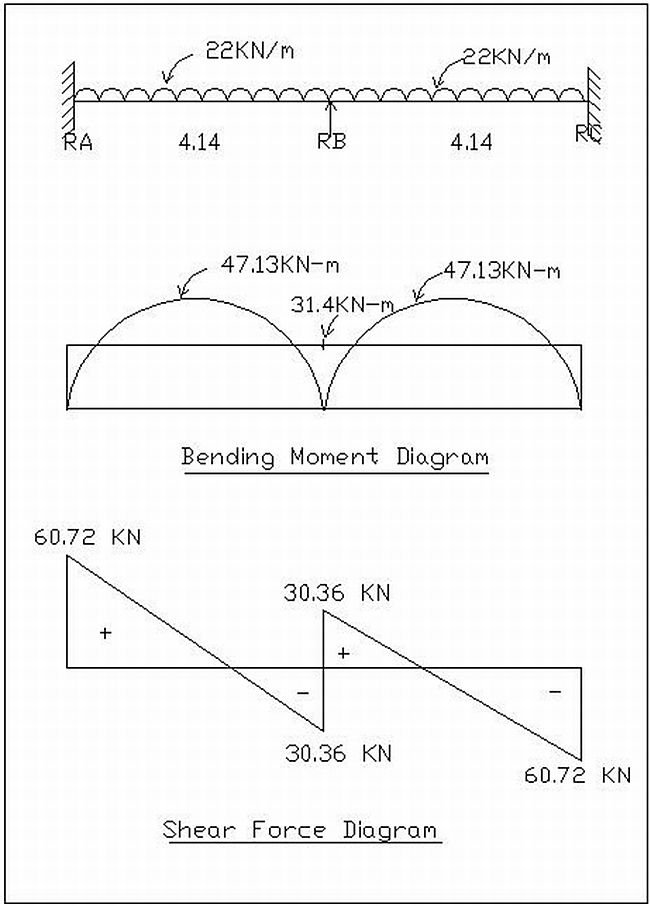## Understanding Load Calculations | Structural Design

#### Load Calculations | Design of Buildings

In our earlier article, we discussed “Different types of loads” and their importance in Structural design.

#### Now we will move on with our further discussion on the following points:

• Design principle assumption and notation assumed
• Design Constant
• Assumptions regarding Design
• Loads on Beams
• Loads on slabs

#### Design principle assumption and notation assumed:

The notations adopted throughout are same as given in IS:456:2000

Density of material used in accordance with reference to IS:857-1987s

 Sr.no Material Density 1 Plain concrete 24 KN/m3 2 Reinforced cement concrete 25 KN/m3 3 Flooring material (cement mortar) 1.00 KN/m3 4 Brick masonry 19 KN/m3

#### Design constant

Using M20 and Fe415 grade of concrete and steel respectively for columns and footings

Therefore:

Fck – i. e. Characteristic strength for M15 – 15 N/mm2

Fck – i. e. Characteristic strength for M15 – 15 N/mm2

Fck – i. e. Characteristic strength for M20 – 20 N/mm2

Fy – i. e. Characteristic strength for steel – 415 N/mm2

## Bending Moment and Fixed Moment Calculations

#### What is Bending Moment?

The element bends when a moment is applied to it. Every structural element has bending moment. Concept of bending moment is very important in the field of engineering especially Civil engineering and Mechanical Engineering.

Unit of measurement: Newton-metres (N-m) or pound-foot or foot-pound (ft.lb)

Bending moment is directly proportional to tensile and compressive stresses. Increase in tensile and compressive stresses results in the increase in the bending moment. These stresses also depend on the second moment of area of the cross section of the element.

#### What is Shear stress?

Shear stress is defined as the measure of force per unit area. Shear stress occurs in shear plane. There are many planes possible at any point in a structure which can be defined to measure stress.

Stress = Force/Unit area

#### Example: Bending Moment and Shear Force CalculationsFrame diagrams | Bending moment and shear force calculations

#### Simply supported bending moment

Mab = wl2/8 = (22×4.14×4.14)/8

= 47.13 KN-m

Mbc = wl2/8 = (22×4.14×4.14)/8

= 47.13 KN-m

Read more

## Calculation of loads for Column and Foundation Design | Structural Design

#### How to calculate the total loads on a column and corresponding footing?

This article has been written on the request from my readers. Engineering students generally get confused when it comes to calculating loads for column and footings design. The manual process is simple.

#### Types of loads on column

1. Self weight of the column x Number of floors
2. Self weight of beams per running meter
3. Load of walls per running meter
4. Total Load of slab (Dead load + Live load + Self weight)

The columns are also subjected to bending moments which have to be considered in the final design. The best way to design a good structure is to use advanced structural design software like ETABS or STAAD Pro. These tools are leagues ahead of manual methodology for structural design, and highly recommended.

In professional practice, there are some basic assumptions we use for structural loading calculations.

You can hire me for your structural design need. Contact me.

For Columns

Self weight of Concrete is around 2400 kg per cubic meter, which is equivalent to 240 kN. Self weight of Steel is around 8000 kg per cubic meter. Even if we assume a large column size of 230 mm x 600 mm with 1% steel and 3 meters standard height, the self weight of column is around 1000 kg per floor, which is equivalent to 10 kN. So, in my calculations, I assume self weight of column to be between 10 to 15 kN per floor.

Read more